# LOG#238. Cosmostuff.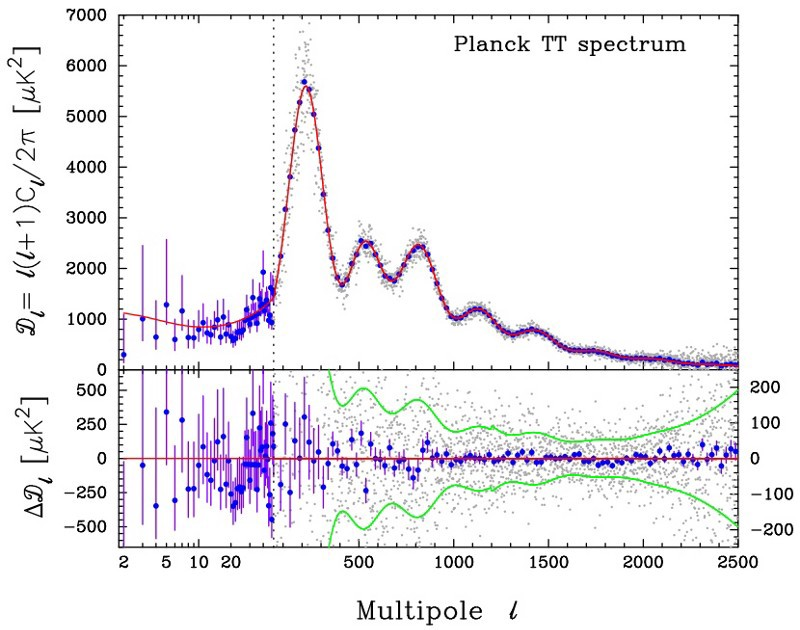Cosmology is facing again some troubles. Some estimations of the Hubble parameter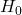differ up to four standard deviations from the accepted value. Even when a few km/s/Mpc are not quite a huge difference, it turns than they can provide anomalies if measured with enough precision.

I am going to review Cosmology today, as far as we know it. It is a quite dynamical field, so changes are expected in the next years with new telescopes, the JWST (James Webb Space Telescope), and other tools or ideas that make this subject so fascinating. After all, isn’t  the Universe as a whole the biggest place where we live? I am neglecting the Multiverse idea as untestable at this moment.

Cosmology can use the so-called standard candles and standard rulers to measure how fast the Universe is expanding. Two measures of distance are

(1)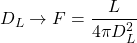(2)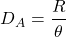and they are called the luminosity distance and the angular distance. They use, respectively, the flux by some strong sources (e.g., SNIa) and the ability to measure the size of an object at long distances (of course, far, far away objects look like point sources, so angular measurement is only possible when having good resolution and/or close enough objects with respect to our instruments).

Considering luminosities, you can measure the bolometric magnitudes:

(3)and

(4)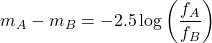Known from the beginning of the second third of the 20th century (Zwicky), we do know that galaxies are not bright enough to explain the galactic motions. The Tully-Fisher relation

(5)and the Faber-Jackson relation

(6)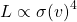are the celebrated luminosities of spiral galaxies and elliptical galaxies, respectively. They hint there are dark matter out there (or equivalently, a component of gravity we do not see with the electromagnetic fluxes).

There are two faint objects we are not sure yet of how many of them are in the galaxies. White dwarfs and neutron stars (I give up the option of black holes and other exotic compact objects, ECOs, at this moment). There are quite strong and robust limitations to the white dwarf and neutron star masses due to nuclear physics. For the former we have the Chandrasekhar limit at about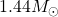, for the latter we have the Tolman-Oppenheimer-Volkoff limit, at about. The uncertainties in the knowledge of the nuclear equation of state for neutron star are the responsible of the relative big mass spreading (1 solar mass). Gravitational waves are going to be the tool to measure the nuclear equation of state, very likely.

The Dark Energy Survey (DES) is a 15000 times 70, i.e., 1050000 dollar project of astronomical Cosmology. PTOLEMY is another future experiment. If there is “no” privileged distance, then we could measure some interesting distances. For instance, a recently new method is the baryonic acoustic oscillations (BAO). What the hell are the BAO? BAO are fluctuations or rapid changes in the density of the visible baryonic matter (normal matter) of the universe, caused by acoustic density waves (changes in the density of baryons, i.e., the proton oscillations at the distances where galaxies are correlated in distance in-and-out) in the primordial plasma of the early universe. The critical acoustic distance is about 150 Mpc or about 500 Mlyr. Then, the correlation function is a device that gives you the probability of two galaxies have to be separated certain distance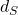. Cosmology, also, measures the rate of expansion of the Universe via a modified Hubble law. Original Hubble law isbut the REAL and more precise Hubble law depends on the redshift(7)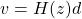In the 21st century, we have more tools to measure what the Universe looks like beyond the electromagnetic visible spectrum. We can handle X-rays, gamma rays, radio waves, neutrinos (extragalactic from 2006 and SN 1987A are known) and gravitational waves (since 2015). Software analysis is diverse: tools like Sextractor or DESI are known in astronomy. General relativity is tested and established as comoslogical theory at the disguise of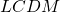. Perfect cosmological principle has been abandonated. We solve the Olbers paradox giving a lifetime of 13.8 Gyrs to our Universe (e.g., see the LSST data and observations, or PLANCK probe ultimate data).  The non-perfect cosmological principle, saying that the Universe is isotropic and homogeneous at distances above 100 Mpc has been tested. GR equations hold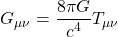with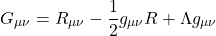Pressure is a source of gravity, through the universal equation of state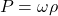There is a critical density to collapse (gravitationally) the Universe. It is given by

(8)or

(9)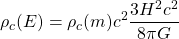Plugging the measured values, it has a value about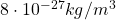, or 10 hydrogen atoms (protons) per cubic meter. Also, you can rewrite that value as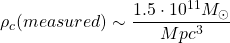Then, knowing, you can define the omega densities as the density of species X over the critical density (dimensionless):The measured value of the cosmological constant energy (or mass) density, is close to the Zeldovich estimate of vacuum energy:This relation has been derived by C. Beck from purely information theory argumentsbeing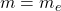the electron mass and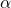the electromagnetic fine structure constant. The Higgs vacuum energy density is much bigger than the measured vacuum energy density, it is about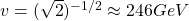, and that is a problem we can not completely understand yet. If so, the Universe should have collapsed. And that is not the case.

In cosmology, theoretical cosmology, we have to measure the redshift in order to know the scale factor of the expansion. They are linked with the equationThe Friedmann equations are only the Einstein Field Equations for a homogeneous and isotropic Universe (described by a metric and a perfect fluid):

(10)(11)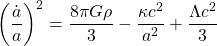(12)Conservation of energy at cosmological scales providesFor matter, dust, we haveand pressureless composition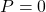. For radiation, we have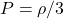and. For dark energy, the cosmological constant Einsteind thought was his biggest mistake, we haveand. Ifwe have the so-called quintessence field. A dynamical field that resembles scalar field but it can also be other classes of field. Quintessence and scalar fields are generally speaking a prediction of superstring theories and other unification models. If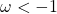we have the so-called phantom energy, a field that could destroy literally the Universe and provide a future singularity. It is the Big Rip (or little Rip in some lite models). There is a relationship between theparameter, the scale factor and the density:

(13)Thus, for dust,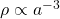, for radiation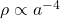andfor dark energy (cosmological constant is constant in density, due to its name of course). Cosmology, at the observational level, tries to fit data to the theory ofvia a global fitof some parameters like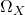,, and other more specific parameters. We can measure the distancewith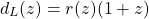The effect of the cosmic expansion is not a Doppler shift. It just enlarges wavelengths. This is the cosmological redshift. It is not a Doppler shift as one usually thinks. Indeed, we have observed objects with redshift above 1, so the relationship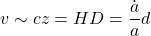implies thatif. Of course, this not implies relativity is wrong. It is only the apparent effect of expansion.

That the Universe suffered a hot dense primordial phase, the Big Bang, is already proved beyond doubts. The COBE satellite and further CMB (cosmic microwave background) probes have even probed that the young Universe was very close to a perfect blackbody body. Indeed, COBE itself proved that at the level of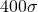. We can now measure the fluctuations of the blackbody CMB temperature in the sky according to some direction. It fits a perfect blackbody with some anisotropies. The power spectrum is the name of the Fourier transform of the temperatura in the sky map. It is measured at the level of some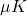, microkelvins. The first peak in that power spectrum fits that our Universe is, surprisingly, flat, since our Universe is close to the critical energy density.implies, thus,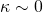. Please, do not tell that to flatlanders! The second peak gives us that there is dark matter out there. There are other hints about that. For instance, the peculiar velocity of galaxies (measured with real Doppler shifts) or the rotational curves of spiral galaxies and the dispersion speed of elliptical galaxies. We expect a cosmic neutrino background at the level ofand a relic graviton background at the level of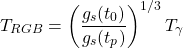If we plug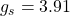as the number of relativistic particle species today, andas the same number evaluated at the Planck time decoupling (that can be only estimated with a GUT/TOE exactly, but we can extrapolate roughly the Standard Model up to that energy to get a bound), you would get arelic graviton background as upper bound (it can be lower if the number of particle species grows at higher energies), so the 1 kelvin degree is a good robust bound. Other theories would lower that value. Extra dimensional theories would also reduce the RGB by modifying the power law of the ratio of the number of particle species.

What else? Dark matter. WIMPs are being testing as candidates since decades ago. They have failed. The naive cross section of Dark Matter would be typically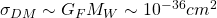. After 25 years of experimental searches, we do know that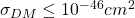, provided it exists of course. WIMPS would have freezed atand annihilation happens with rates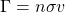. Relativistic dark matter are constrained by the number of known light neutrino species, not being radiation! No experiment has detected dark matter particles yet. Anyway, it could be anything between about 80 magnitude orders.

Finally, have you ever wondered how many eras in the cosmic evolution have the Universe faced? Let me list them:

1. Definition 1 (Luminosity distance).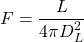.
1. The Planck era: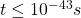. Quantumg gravity is necessary here, and likely the next two or three eras.
2. The inflation era: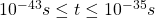. Inflation, exponential expanding Universe, was suffered her. Otherwise, the flatness problem and the observed structure could be impossible. B-modes in the CMB would be a proof of inflation. After BICEP2 blunder, we have to wait for further evidences.
3. Leptogenesis and baryogenesis. Where do quark and leptons come from? Why and how were they formed? We do not know, but this era is a future target of some experiments and theoretical studies. If neutrinos were Majorana particles or Majorana states for the neutrino species (right-handed!) wher found, we could explain, in principle, the unbalance between matter and antimatter we observe today.
4. Electroweak and QCD phase transitions. In the edge of our current knowledge thanks to ALICE and quark-gluon plasma studies and the discovery of the Higgs field permeating the Universe. Further studies of this era will require gravitational waves and neutrinos, since no photon can show us information of this a previous dark eras.
5. Neutrino decoupling. At about 1 second from the Big Bang, neutrino decouples from the primordial plasma.
6. Electron-positron annihilation. After neutrino decoupled, electron and positros begin annihilating.
7. Big Bang nucleosynthesis. The Universe is cooling and at some point, first nuclei (hydrogen, helium and lithium) confine. The point where matter and radiation pressures equalize is found. It happens at about 3 minutes. The cosmic temperature is about 3000 K, and it is lower than the 158000 K of the 13.6 eV ground state of the hydrogen atom.
8. Recombination. The Universe expands and cools further. After 380000 years, electrons bound to the first atoms (H, He and Li). Big Bang theory predict the relative abundances of the main isotopes. The first stars (Population III) will form in the next millions of years, breeding the Universe with black hole seeds and galaxies after billions of years (Gyrs).
9. At some point, a few Gyrs ago, dark energy begins dominate the cosmic expansion, previously dominated by matter. Thus, from radiation dominated expansion, we passed a several Gyr era of matter dominated expansion until we end in this dark energy era. Is emergent life related to dark energy dominating the cosmic expansion? Probably not, but it is a striking coincidence.

See you in the next blog post!

Definition 2 (Angular distance)..

Definition 3 (Friedmann equations).Definition 4 (Perfect fluid energy-momentum tensor).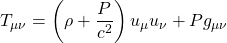.

Definition 5 (Cosmic neutrino and graviton backgrounds).This site uses Akismet to reduce spam. Learn how your comment data is processed.